## *SPECIFIC GAS CONSTANT

Keyword type: model definition, material

With this option the specific gas constant of a material can be defined. The specific gas constant is required for a calculation in which a gas dynamic network is included. The specific gas constant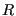is defined as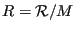(648)

where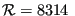J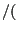kmol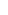K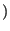is the universal gas constant and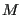is the molecular weight of the material. The specific gas constant is temperature independent.

First line:

• *SPECIFIC GAS CONSTANT

Following line:

• Specific gas constant.

Example:

*SPECIFIC GAS CONSTANT
287.


defines a specific gas constant with a value of 287. This value is appropriate for air if Joule is chosen for the unit of energy, kg as unit of mass and K as unit of temperature, i.e.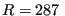JkgK.

Example files: linearnet, branch1, branch2.